Publicité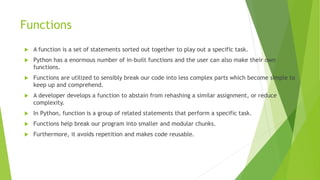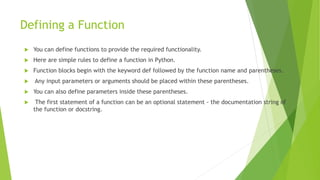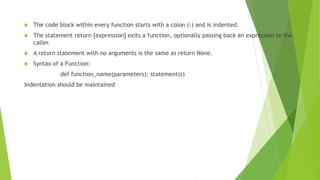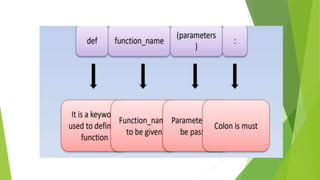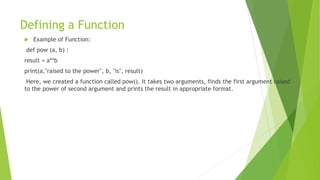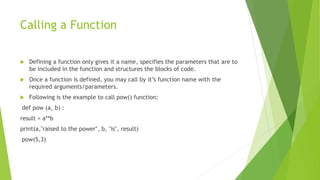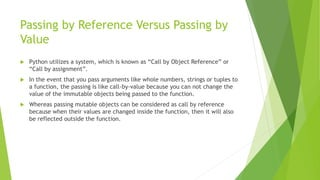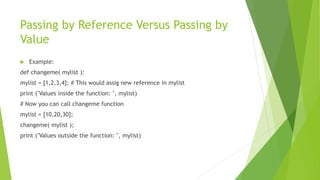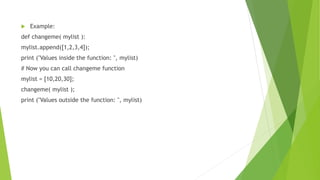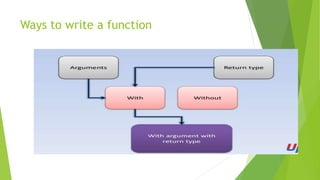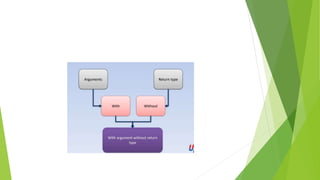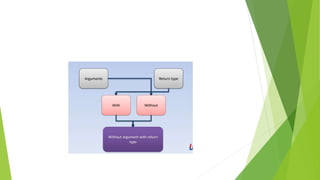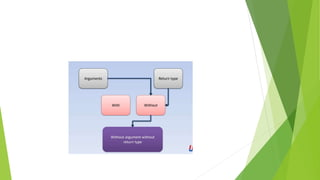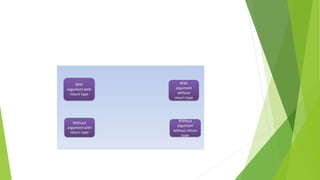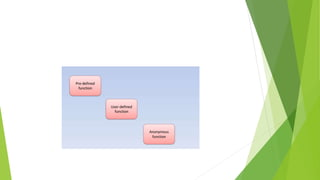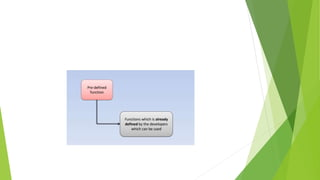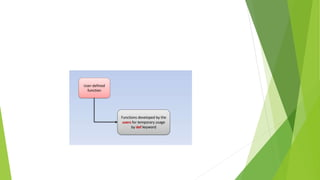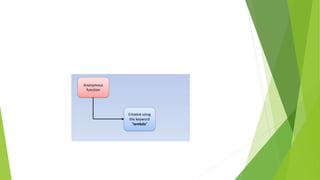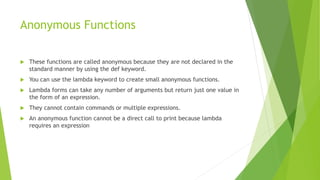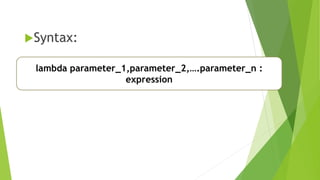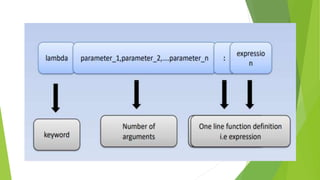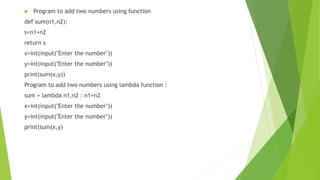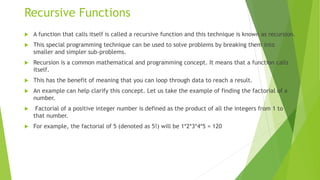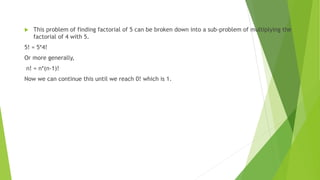1 sur 27
Publicité

### functions.pptx

1. FUNCTIONS
2. Learning outcomes:  What is Function?  Defining a Function  Calling a Function  Passing by Reference vs Passing by Value  Ways to write a function  Types of functions  Anonymous Functions  Recursive Function
3. Functions  A function is a set of statements sorted out together to play out a specific task.  Python has a enormous number of in-built functions and the user can also make their own functions.  Functions are utilized to sensibly break our code into less complex parts which become simple to keep up and comprehend.  A developer develops a function to abstain from rehashing a similar assignment, or reduce complexity.  In Python, function is a group of related statements that perform a specific task.  Functions help break our program into smaller and modular chunks.  Furthermore, it avoids repetition and makes code reusable.
4. Defining a Function  You can define functions to provide the required functionality.  Here are simple rules to define a function in Python.  Function blocks begin with the keyword def followed by the function name and parentheses.  Any input parameters or arguments should be placed within these parentheses.  You can also define parameters inside these parentheses.  The first statement of a function can be an optional statement - the documentation string of the function or docstring.
5.  The code block within every function starts with a colon (:) and is indented.  The statement return [expression] exits a function, optionally passing back an expression to the caller.  A return statement with no arguments is the same as return None.  Syntax of a Function: def function_name(parameters): statement(s) Indentation should be maintained
6. Defining a Function  Example of Function: def pow (a, b) : result = a**b print(a,"raised to the power", b, "is", result) Here, we created a function called pow(). It takes two arguments, finds the first argument raised to the power of second argument and prints the result in appropriate format.
7. Calling a Function  Defining a function only gives it a name, specifies the parameters that are to be included in the function and structures the blocks of code.  Once a function is defined, you may call by it’s function name with the required arguments/parameters.  Following is the example to call pow() function: def pow (a, b) : result = a**b print(a,"raised to the power", b, "is", result) pow(5,3)
8. Passing by Reference Versus Passing by Value  Python utilizes a system, which is known as “Call by Object Reference” or “Call by assignment”.  In the event that you pass arguments like whole numbers, strings or tuples to a function, the passing is like call-by-value because you can not change the value of the immutable objects being passed to the function.  Whereas passing mutable objects can be considered as call by reference because when their values are changed inside the function, then it will also be reflected outside the function.
9. Passing by Reference Versus Passing by Value  Example: def changeme( mylist ): mylist = [1,2,3,4]; # This would assig new reference in mylist print ("Values inside the function: ", mylist) # Now you can call changeme function mylist = [10,20,30]; changeme( mylist ); print ("Values outside the function: ", mylist)
10.  Example: def changeme( mylist ): mylist.append([1,2,3,4]); print ("Values inside the function: ", mylist) # Now you can call changeme function mylist = [10,20,30]; changeme( mylist ); print ("Values outside the function: ", mylist)
11. Ways to write a function
12. Anonymous Functions  These functions are called anonymous because they are not declared in the standard manner by using the def keyword.  You can use the lambda keyword to create small anonymous functions.  Lambda forms can take any number of arguments but return just one value in the form of an expression.  They cannot contain commands or multiple expressions.  An anonymous function cannot be a direct call to print because lambda requires an expression
13. Syntax: lambda parameter_1,parameter_2,….parameter_n : expression
14.  Program to add two numbers using function def sum(n1,n2): s=n1+n2 return s x=int(input("Enter the number")) y=int(input("Enter the number")) print(sum(x,y)) Program to add two numbers using lambda function : sum = lambda n1,n2 : n1+n2 x=int(input("Enter the number")) y=int(input("Enter the number")) print(sum(x,y)
15. Recursive Functions  A function that calls itself is called a recursive function and this technique is known as recursion.  This special programming technique can be used to solve problems by breaking them into smaller and simpler sub-problems.  Recursion is a common mathematical and programming concept. It means that a function calls itself.  This has the benefit of meaning that you can loop through data to reach a result.  An example can help clarify this concept. Let us take the example of finding the factorial of a number.  Factorial of a positive integer number is defined as the product of all the integers from 1 to that number.  For example, the factorial of 5 (denoted as 5!) will be 1*2*3*4*5 = 120
16.  This problem of finding factorial of 5 can be broken down into a sub-problem of multiplying the factorial of 4 with 5. 5! = 5*4! Or more generally, n! = n*(n-1)! Now we can continue this until we reach 0! which is 1.
17. Thank you
Publicité